# Rate law and specific rate constant

Chemical Kinetics: Rate law and specific rate constant

A rate law is an expression shows the relationship between the reaction rate and the concentrations of each reactant in a system.

For any general reaction at a fixed temperature

aA + bB → cC + dD

Rate = k[A]m[B]n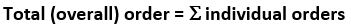The specific rate constant (k) is the proportionality constant that specifically shows the relationship between the rate and the concentrations of reactants. Specific rate constant is independent of concentration but still dependent on temperature and mechanism of the reaction. The rate law exponent or order (m and n) and the specific rate constant for any chemical reaction must be determined experimentally. The values of m and n are not related to the coefficients a and b since m and n are obtained experimentally. The high value of the rate constant shows that the reaction is relatively fast, while a low value of the rate constant indicates that the reaction is relatively slow.

To determine the rate constant and other parameters, one must collect data using different concentrations of reactants. Simple experiments are done by doubling 1 concentration value at a time and looking at the effect.

 order doubling effect on the rate 0 0 = 1 none 1/2 1/2 = 1.41.. increase by 1.41 1 1 = 2 doubles 2 2 = 4 quadruplesFig 1:Information sequence to determine the kinetic parameters of a reaction.

Consider the following example:the following data were obtained experimentally for the initial rates disappearance of NO: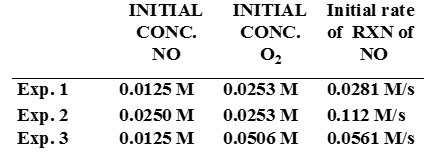Overall rate expression: rate = k[NO]x[O2]y

For NO: select a pair of experimental values where the concentration of [NO] is changed, but other concentrations remain unchanged.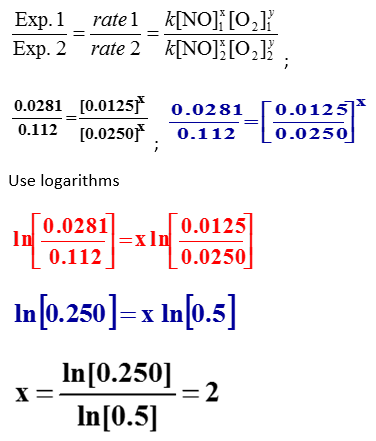For O2: select a pair of experimental values where the concentration of [O2] is changed, but all other concentrations remain unchanged. Use experiment 1 and 3 for further analysisAn integrated rate law includes time as a variable while a half-life is a time taken for the concentration of a reactant to drop to half its original value. In other words, the half-life, t1/2 is the time required for [A]0 to reach ½[A]0.

 Zero Order First Order Second Order Rate law rate = k rate = k[A] rate = k[A]2 Units for k mol/L·s 1/s L/mol·s Half-life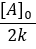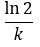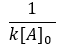Integrated rate law Straight line format [A]t = –kt + [A]0 ln[A]t = –kt + ln[A]0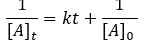Plot for straight line [A]t vs. t ln[A]t vs. t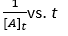Slope, y intercept –k, [A]0 -k, ln[A]0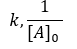Plot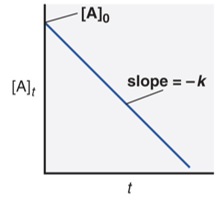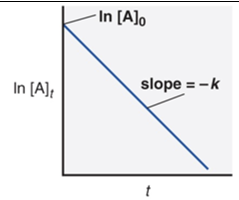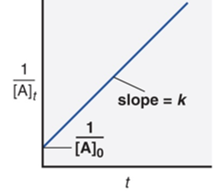Figure 2: [A] is the concentration of A at any time t and [A]0 is the concentration of A at time t=0

The rate-determining or rate-limiting step is defined as the slowest step in a reaction. The following reaction has been proposed to occur by a two-step mechanism:

NO2(g) + CO(g) → NO(g) + CO2(g)

NO2(g) + NO2(g) → NO3(g) + NO(g) [slow reaction; rate-determining step]

(2NO2(g) + CO(g) → NO2(g) + CO2(g) [fast reaction]

Observed rate law: rate = k[NO2]2

Hence, the rate law for the rate-determining step can be written as the rate law for the overall reaction.